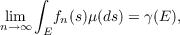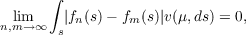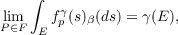#### Vol. 23, No. 1, 1967

 Download this articleFor screen For printingRecent Issues Vol. 325: 1 Vol. 324: 1  2 Vol. 323: 1  2 Vol. 322: 1  2 Vol. 321: 1  2 Vol. 320: 1  2 Vol. 319: 1  2 Vol. 318: 1  2Online Archive Volume: Issue:The Journal Subscriptions Editorial Board Officers Contacts Submission Guidelines Submission Form Policies for Authors ISSN: 1945-5844 (e-only) ISSN: 0030-8730 (print) Special Issues Author Index To Appear Other MSP Journals

### Charles L. Fefferman

Vol. 23 (1967), No. 1, 35–45
##### Abstract

Suppose that Σ is a field of subsets of the set S, and suppose that μ and γ are complex-valued finitely additive set functions defined on Σ. Assume that μ is bounded and γ is finite and absolutely continuous with respect to μ. (A word of warning is in order here. The statement γ is absolutely continuous with respect to μ” is often interpreted as “μ(E) = 0 implies γ(E) = 0”. This is not the meaning used here. Our definition is “for every 𝜖 > 0 there is a δ > 0 such that |β(E)| < δ implies |γ(E)| < 𝜖.” Unless μ is bounded and countably additive, the two definitions are not equivalent.)

THEOREM 1. There exists a sequence {fn} of μ-simple functions on S, such that(1)

uniformly for E Σ(2)

where v(μ) is the total variation of μ.

Theorem 1 is established by a pure existence proof, and gives no indication of how to find fn. A more constructive result is given below.

A partition of S is a finite collection of sets Ei belonging to Σ, such that S is the disjoint union of the Ei, and such that μ(Ei)0,i = 1,,n.

The set 𝒫 of partitions may be directed by refinement, that is, by the following partial order: P1 < P2 if for every E P1 there exist F1,,Fr P2 ( r may depend on E) such that E and i=1rFi differ by a μ-null set.

If P is a partition of S, define the μ-simple function fpγ to be EP(γ(E)∕μ(E))χE, where χE is the characteristic function of E.

THEOREM 2. If ∕ℓ is positive, thenuniformly for E Σ, where 𝒫 is directed as explained above.

Primary: 28.16
##### Milestones